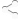Welcome to Obsidian Forum Community# How Accuracy is So Important in DMG

Accuracy DPS

### #1Kagemitsu Posted 07 January 2019 - 09:16 PM

Kagemitsu

(0) Nub

•• Initiates
• 4 posts

First of all, I'd like to say that im still new on this game but im so amazed on the game mechanics that i cant help but to create my own DPS calculator.

There are so many factor which affects the DPS but for now id like to discuss how Accuracy stat really affects the DPS outcome of your character.

(Any criticism is very welcome, you can contest your own opinion if you want. It would help me also have a deeper insight for the game.)

In my understanding, accuracy determines the success rate of your attacks, regardless of how high your DMG if you dont land a hit then its close to useless.

Success Rate =  (Accuracy - Deflection) + (1-100 Roll)

If the sum is:

1-15 = Miss - You have a 15% chance that you will never land a hit.

16-50 = Graze - 35% chance, you will land a hit but it will be only 50% of your DMG (0.5DMG)

51-100 = Hit - 50% chance to land 100% of your DMG.

>100 = 0% chance, but if you land it it will be x1.5DMG(Awesome!)

Now lets assume that your Average weapon DMG is 17 (14-20 for Great Swords), for starters. And you are a level 1 Fighter (Accuracy=30) fighting a young wolf with 24 Deflection,

your total accuracy before Roll is 6(30-24=6). giving you a:

(6 + 1) - 15 = 7-15 = 9% Miss chance

16 - 50 =                   35% Graze chance

51-100 =                   50% Hit Chance

101 - 106 =               6% Crit Chance.

Multiplying your DMG to the percentage would give you your Average DPS.

Percentage x DMG Multiplier  x  Ave DMG    Total

-----------------------------------------------------------------------

0.09 x 0.0 x 17 = 0

0.35 x 0.5 x 17 = 2.975

0.50 x 1.0 x 17 = 8.5

0.06 x 1.5 x 17 = 1.53

Total DMG = 0 + 2.975 + 8.5 + 1.53 = 13.005 or  approx. 13

To prove the basis of calculation, Suppose to be you have a 100% chance to always land a hit, (1 x 1 x 17= 17) that would give you a full potential of your weapon damage since its always a hit.

And if you had only a 50% chance of hit and 50% on crit(say because you had an overwhelming Accuracy), the calculation would be:

(0.5 x 1 x 17) + (0.5 x 1.5 x 17) = (8.5) + (12.75) = 21.25

which is gives you 25% more of your weapon potential.(Amazing)

Now at Level 1 Fighter I always choose the "Disciplined Barrage" over "Knock Down" because of the bonus + 20 Accuracy. Calculating the added accuracy of the ability would give me a DMG of:

Accuracy before Roll = (30 + 20 -24) = 26

Miss = 0%

Graze (27-50) = 24%

Hit (51-100) = 50%

Crit (101-126) = 26%

therefore:

0.00 x 0.0 x 17 = 0

0.24 x 0.5 x 17 = 2.04

0.50 x 1.0 x 17 = 8.5

0.26 x 1.5 x 17 = 6.63

Total DMG = 0 + 2.04 + 8.5 + 6.63 = 17.17 DMG before Damage Reduction.

Now I know that there are a lot of factor affecting the DMG,(Might,Dex, equipment etc) but i guess Accuracy is as important as other stats.

Now Im trying to make a DPS calculator, and im reading the Weapon attack speed from other forums to do this so.

What are your thoughts guys.

### Also tagged with one or more of these keywords: Accuracy, DPS

#### 0 user(s) are reading this topic

0 members, 0 guests, 0 anonymous users